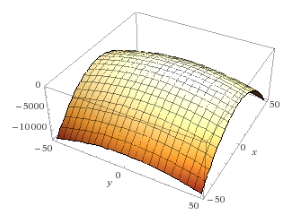# Find fx(1, 0) and fy(1, 0) and interpret these numbers as slopes for the following equation

If f(x,y)=16-4x^2-y^2, find fx(1,2) and fy(1,2) and interpret these numbers as slopes. Illustrate with either hand-drawn sketches or computer plots.